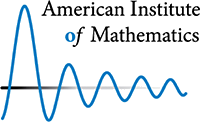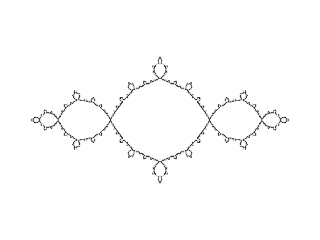### Highlights#### The quest for uniform bounds

AIM - April 2022Understanding what happens when one iterates the values of a function can lead to some interesting mathematics.
For the simplest example consider the quadratic function f(z) = z^2 - 1.  Starting with z = 2, we get f(2) = 3. then f(3) = 8, f(8) = 63, and so on. It is clear the iterates are unbounded. Something different happens starting with z = 1/2. In this case the values do not grow. They always stay between -1 and 1. And starting with z = 0, we have f(0) = -1, f(-1) = 0, f(0) = -1, so that the values alternate between 0 and 1. The numbers 0 and -1 are periodic points for the function f(z)=z^2-1.

Now consider two such functions, say f(z) = z^2 + a and f(z) = z^2 + b, where the a and b are different complex numbers. One ask if they can have any periodic points in common? And if they do, how many common ones can there be? The surprising answer to this is that, regardless of the values of a and b, the number of common periodic points is always less than some fixed number M, which is known to be at least 5. Just how large is M? That remains a tantalizing question.

A much more difficult problem, but one with some of the same flavor, occurs in the study of certain surfaces of genus two in trying to understand how each sits inside a 4-dimensional torus, called its Jacobian. There is a large set of special points in this Jacobian, the "torsion'' points. In the early 1980s, Raynaud proved that the surface will intersect only finitely many of these points, which had been conjectured earlier by Manin and Mumford. Soon thereafter, Mazur posed an important question about these surfaces, asking if we can bound the number of the special points on the surface only in terms of its genus (the topology of the surface), or if there is additional geometric information needed to control the size of this intersection.

Although not obviously related to number theory, much progress on questions of this sort had been made over the years with tools from algebra and arithmetic geometry. But the answer to Mazur's question about a uniform bound remained elusive.

In the paper, "Uniform Manin-Mumford for a family of genus 2 curves,'' the authors Laura DeMarco, Holly Krieger, and Hexi Ye employ arithmetic tools and ideas from dynamical systems to finally provide a uniform bound on the number of torsion points. They study a family of surfaces in genus 2 that map to pairs of elliptic curves (surfaces of genus 1), and they give a positive answer to Mazur's question for this family. The proofs employ a quantitative version of arithmetic equidistribution to relate the heights (i.e., a measure of the arithmetic complexity) of the surfaces to the number of torsion points.

This paper appeared in 2021 in the Annals of Mathematics and grew out of the AIM SQuaRE, Dynamical Andre-Oort Questions. The authors received the 2020 Alexanderson Award which recognizes outstanding research articles arising from AIM research activities that have been published within the past three years.